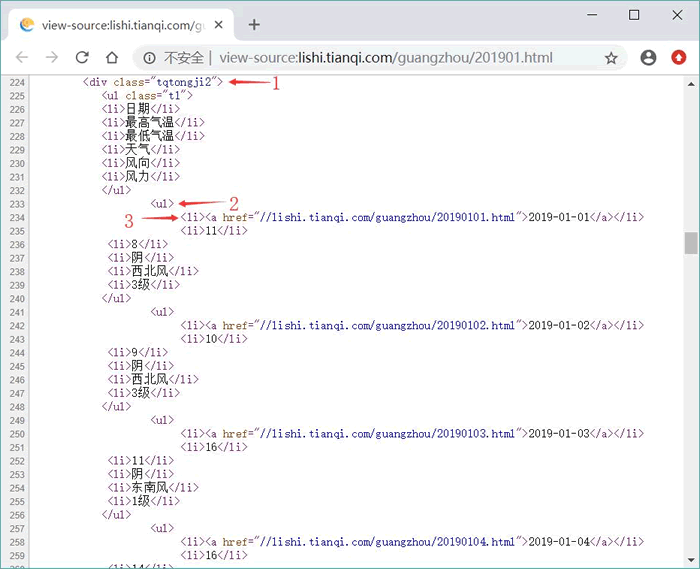# Python读取网络数据```import re
from datetime import datetime
from datetime import timedelta
from matplotlib import pyplot as plt
from urllib.request import *
# 定义一个函数读取lishi.tianqi.com的数据
def get_html(city, year, month):  #①
url = 'http://lishi.tianqi.com/' + city + '/' + str(year) + str(month) + '.html'
# 创建请求
request = Request(url)
# 添加请求头
'AppleWebKit/537.36 (KHTML, like Gecko) Chrome/54.0.2840.99 Safari/537.36')
response = urlopen(request)
# 获取服务器响应
# 定义3个list列表作为展示的数据
dates, highs, lows = [], [], []
city = 'guangzhou'
year = '2017'
months = ['01', '02', '03', '04', '05', '06', '07',
'08', '09', '10', '11', '12']
prev_day = datetime(2016, 12, 31)
# 循环读取每个月的天气数据
for month in months:
html = get_html(city, year, month)
# 将html响应拼起来
text = "".join(html.split())
# 定义包含天气信息的div的正则表达式
patten = re.compile('<divclass="tqtongji2">(.*?)</div><divstyle="clear:both">')
table = re.findall(patten, text)
patten1 = re.compile('<ul>(.*?)</ul>')
uls = re.findall(patten1, table)
for ul in uls:
# 定义解析天气信息的正则表达式
patten2 = re.compile('<li>(.*?)</li>')
lis = re.findall(patten2, ul)
# 解析得到日期数据
d_str = re.findall('>(.*?)</a>', lis)
try:
# 将日期字符串格式化为日期
cur_day = datetime.strptime(d_str, '%Y-%m-%d')
# 解析得到最高气温和最低气温
high = int(lis)
low = int(lis)
except ValueError:
print(cur_day, '数据出现错误')
else:
# 计算前、后两天数据的时间差
diff = cur_day - prev_day
# 如果前、后两天数据的时间差不是相差一天，说明数据有问题
if diff != timedelta(days=1):
print('%s之前少了%d天的数据' % (cur_day, diff.days - 1))
dates.append(cur_day)
highs.append(high)
lows.append(low)
prev_day = cur_day
# 配置图形
fig = plt.figure(dpi=128, figsize=(12, 9))
# 绘制最高气温的折线
plt.plot(dates, highs, c='red', label='最高气温',
alpha=0.5, linewidth = 2.0)
# 再绘制一条折线
plt.plot(dates, lows, c='blue', label='最低气温',
alpha=0.5, linewidth = 2.0)
# 为两个数据的绘图区域填充颜色
plt.fill_between(dates, highs, lows, facecolor='blue', alpha=0.1)
# 设置标题
plt.title("广州%s年最高气温和最低气温" % year)
# 为两条坐标轴设置名称
plt.xlabel("日期")
# 该方法绘制斜着的日期标签
fig.autofmt_xdate()
plt.ylabel("气温（℃）")
# 显示图例
plt.legend()
ax = plt.gca()
# 设置右边坐标轴线的颜色（设置为none表示不显示）
ax.spines['right'].set_color('none')
# 设置顶部坐标轴线的颜色（设置为none表示不显示）
ax.spines['top'].set_color('none')
plt.show()```Python教程

## Python functools模块完全攻略

2021-4-27 7:47:27

Python教程

## Python __dir__用法：列出对象的所有属性（方法）名

2021-4-27 7:47:39

0 条回复 A文章作者 M管理员
暂无讨论，说说你的看法吧